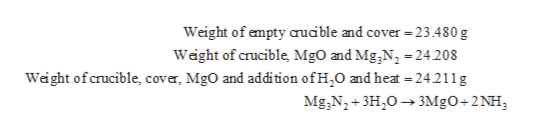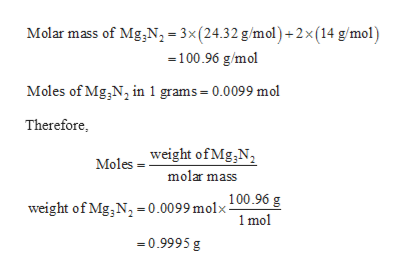# Mg3N2 +3H2O -> 3MgO+2NH3 Weight of empty crucible and cover :   23.480 gWeight of crucible, cover, MgO and Mg3N2 :   24.208 gWeight of crucible, cover, MgOand addition of H2O and heat :   24.211 g Calculate the change in mass.Calculate the number of moles of Mg3N2. Calculate the number of grams of Mg3N2. Calculate the weight % of Mg3N2. Calculate the weight % og MgO

Question
22 views

Mg3N2 +3H2O -> 3MgO+2NH3

Weight of empty crucible and cover :   23.480 g

Weight of crucible, cover, MgO and Mg3N2 :   24.208 g

Weight of crucible, cover, MgOand addition of H2O and heat :   24.211 g

Calculate the change in mass.

Calculate the number of moles of Mg3N2.

Calculate the number of grams of Mg3N2.

Calculate the weight % of Mg3N2.

Calculate the weight % og MgO

check_circle

Step 1

The data are given as follows:help_outlineImage TranscriptioncloseWeight of empty aucible and cover 23.480 g Weight of crucible, MgO and Mg,N2 =24.208 Weight ofcrucible, cover, MgO and addition ofH20 and heat - 24211g Mg,N2+3H203MgO+2NH; fullscreen
Step 2

The change in mass can be calculated as follows:

Step 3

Weight of Mg3N2 can be calculated...help_outlineImage TranscriptioncloseMolar mass of Mg,N, =3x(24.32 g/mol)+2x (14 g/mol) 100.96 g/mol Moles of Mg,N2 in 1 grams - 0.0099 mol Therefore Moles - weight ofMg,N, molar mass = 0.0099 molx100.96 g 1 mol weight of Mg,N 0.9995 g fullscreen

### Want to see the full answer?

See Solution

#### Want to see this answer and more?

Solutions are written by subject experts who are available 24/7. Questions are typically answered within 1 hour.*

See Solution
*Response times may vary by subject and question.
Tagged in

### Chemistry